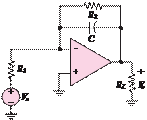### Create an Account

Home / Questions / 863 The inverting amplifier shown in Figure P863 can be used as a low pass filter a De...

# 863 The inverting amplifier shown in Figure P863 can be used as a low pass filter a Derive the frequency response of the circuit

8.63 The inverting amplifier shown in Figure P8.63 can be used as a low-pass filter.

a.    Derive the frequency response of the circuit.

b.    If R1 = R2 = 100 kand = 0.μF, compute the attenuation in decibels at ω = 1,000 rad/s.

c.    Compute the gain and phase at ω = 2,500 rad/s.

d.    Find the range of frequencies over which the attenuation is less than 1 decibel.R1

Vin

C     R2Jun 18 2020 View more View LessSubscribe To Get Solution